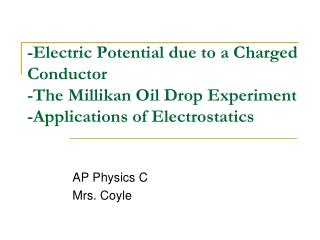DownloadDownload Presentation-Electric Potential due to a Charged Conductor -The Millikan Oil Drop Experiment -Applications of Electrostatics

# -Electric Potential due to a Charged Conductor -The Millikan Oil Drop Experiment -Applications of Electrostatics

Télécharger la présentation## -Electric Potential due to a Charged Conductor -The Millikan Oil Drop Experiment -Applications of Electrostatics

- - - - - - - - - - - - - - - - - - - - - - - - - - - E N D - - - - - - - - - - - - - - - - - - - - - - - - - - -
##### Presentation Transcript

1. -Electric Potential due to a Charged Conductor-The Millikan Oil Drop Experiment-Applications of Electrostatics AP Physics C Mrs. Coyle

2. Electric Potential –What we used so far! • Electric Potential • Potential Difference • Potential for a point charge • Potential for multiple point charges • Potential for continuous charge distribution

3. Is the surface of a charged conductor an equipotential? • Is the electric potential constant everywhere inside a charged conductor and equal to its value at the surface?

4. Electric Potential Difference on the Surface of a Charged Conductor in Equilibrium • Let A and B be points on the surface of the charged conductor • Let ds be the displacement from A to B. • E is always perpendicular to the displacement ds. So, E ·ds = 0 • Therefore, the potential difference between A and B is also zero

5. Electric Potential Difference on the Surface of a Charged Conductor in Equilibrium • V is constant everywhere on the surface of a charged conductor in equilibrium • ΔV = 0 between any two points on the surface • The surface of any charged conductor in electrostatic equilibrium is an equipotential surface

6. What about the inside of a charged conductor? • E=0 inside the conductor in equilibrium • E ·ds = 0 • Therefore, the electric potential is constant everywhere inside the conductor and equal to the value at the surface.

7. Solid Conducting Sphere • r<R V=kq/R E=0 • r=R V=kq/R E=kQ/R2 • r>R V=kq/r E=kQ/r2 Note: • V is a Scalar related to energy • E is a Vector related to force.

8. Irregularly Shaped Conductors • The charge density is high where the radius of curvature is small • The electric field is high at sharp points

9. Irregularly Shaped Conductors • The field lines are perpendicular to the conducting surface • The equipotential surfaces are perpendicular to the field lines

10. Quick Quiz 25.10 Draw a graph of the electric potential as a function of position relative to the center of the left sphere. (Left sphere 1, radius a),(Right sphere 2, radius c) The centers of the spheres are a distance b apart.

11. Quick Quiz 25.10 Answer: See below. Notice the flat plateaus at each conductor, representing the constant electric potential inside a solid conductor.

12. Ex 25.9: Two Connected Charged Spheres • The separation distance of the spheres is much greater than the radius of either sphere so their fields do not affect each other. • Show

13. ΔV= O in a Cavity in a Conductor, so Equipotential to Body of Conductor • Assume no charges are inside the cavity • E=0 inside the conductor • The electric field inside does not depend on the charge distribution on the outside surface of the conductor

14. Corona Discharge • If the electric field near a conductor is sufficiently strong, electrons resulting from random ionizations of air molecules near the conductor accelerate away from their parent molecules • These electrons can ionize additional molecules near the conductor

15. Corona Discharge • The glow that is observed near a charged conductor of a strong E-field. • It results from the recombination of freed electrons with the ionized air molecules • Most likely to occur near very sharp points

16. Millikan Oil-Drop Experiment

17. Millikan Oil-Drop Experiment • Robert Millikan measured e, the charge of the electron e = 1.60 x 10-19 C • He also demonstrated the quantized nature of this charge

18. Oil-Drop Experiment • With no electric field between the plates: The drop reaches terminal velocity with FD = mg

19. Oil-Drop Experiment • When an electric field is set up between the plates the drop moves upwards and reaches a new terminal velocity • Fe = mg +FD =qE • Solve for q • Observed thousands of times and always found e=multiple of 1.6x10-19 C

20. Van de Graaff Generator

21. Electrostatic PrecipitatorOn Off

22. Electrostatic Precipitator • It removes particulate matter like ashes from combustion gases • Corona discharge ionizes particles of air • Most of the dirt particles are negatively charged and are drawn to the walls by the electric field of the negative potential coil.